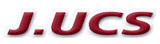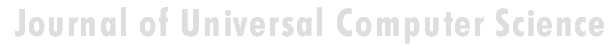Search
 Submission Procedure share: |available in:   PDF (147 kB) PS (47 kB)

get:
 Similar Docs BibTeX Write a comment
get:

DOI:   10.3217/jucs-003-10-1121

### A Note on Correctness Proofs for Overflow Detection Logic in Adders for d-th Complement Numbers

Bernd Rederlechner (Telekom Entwicklungszentrum Südwest, Germany)

Jörg Keller

Abstract: When adding n-bit 2-th complement numbers, the result can be outside the range representable with n bits. A well-known theorem justifies the common overflow logic: Let a,b{0,1}n be the 2-th complement representations of signed integers [a] and [b], respectively, and let c0{0, 1} be the carry-in bit. Then, [a] + [b] + c0{-2n-1,...,2n-1-1} if and only if cn = cn-1 , where ci denotes the carry-bit from position i - 1 to position i when adding the binary numbers a and b. We present a proof of this theorem which is much shorter than previous proofs. This simplification can save valuable time in computer science classes. With a small extension the proof even holds for d-th complement numbers. Although the proof technique is known by some specialists, nobody seems to have written it up. With this note, it is once documented in a precise form, thus avoiding re-invention.

Keywords: computer science education, correctness proof, d-ary arithmetic, overflow testing

Categories: B.2, K.3.2